Function Repository Resource:

# OsculatingConic

Get the osculating conic of a plane curve

Contributed by: Jan Mangaldan
 ResourceFunction["OsculatingConic"][c,{t,t0},{x,y}] gives the implicit equation with variables x and y for the osculating conic of a plane curve c depending on parameter t at t=t0.

## Details

The osculating conic of a plane curve at a given point is the unique conic that has fourth-order contact with the curve at that point.

## Examples

### Basic Examples (2)

The implicit Cartesian equation for the osculating conic of a deltoid:

 In:=Out=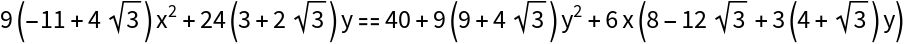Plot the deltoid and its osculating conic along with their point of contact:

 In:=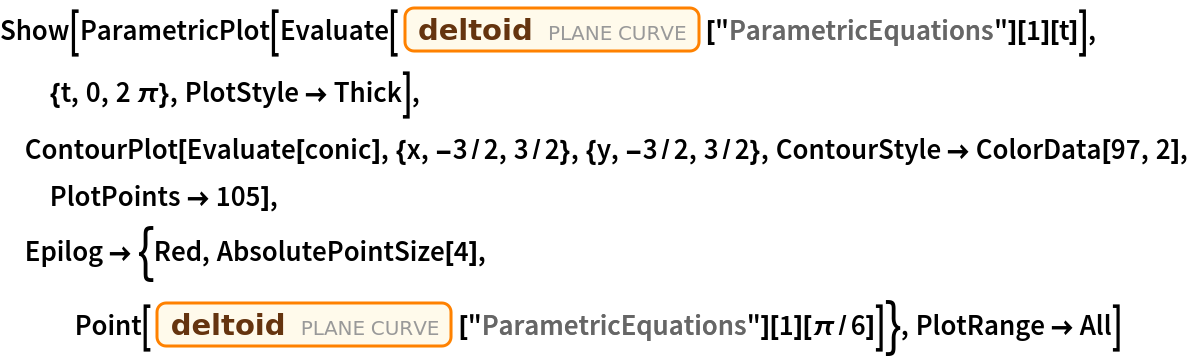Out=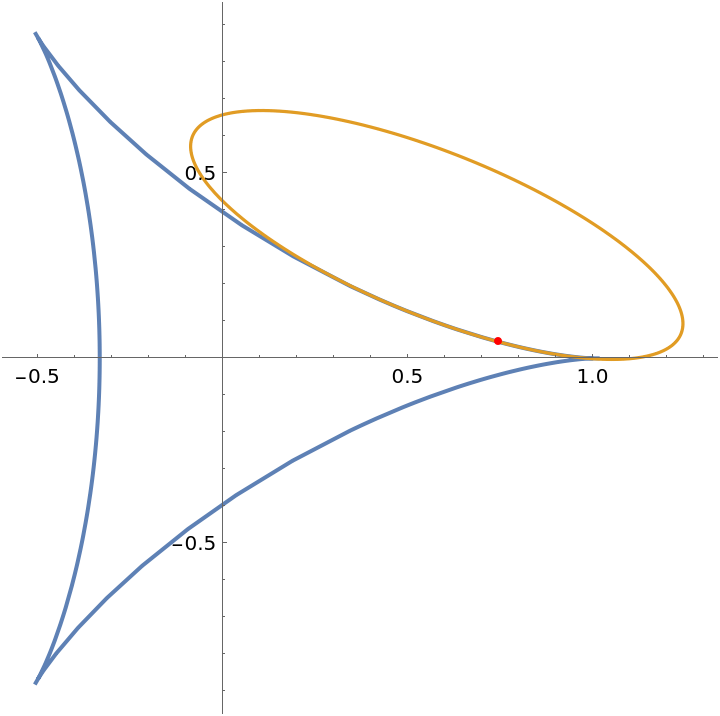### Scope (2)

The osculating conic for a catenary:

 In:=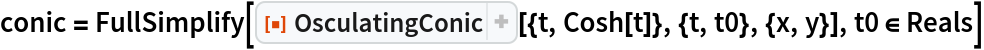Out=Show three of the osculating conics along with the catenary:

 In:=Out=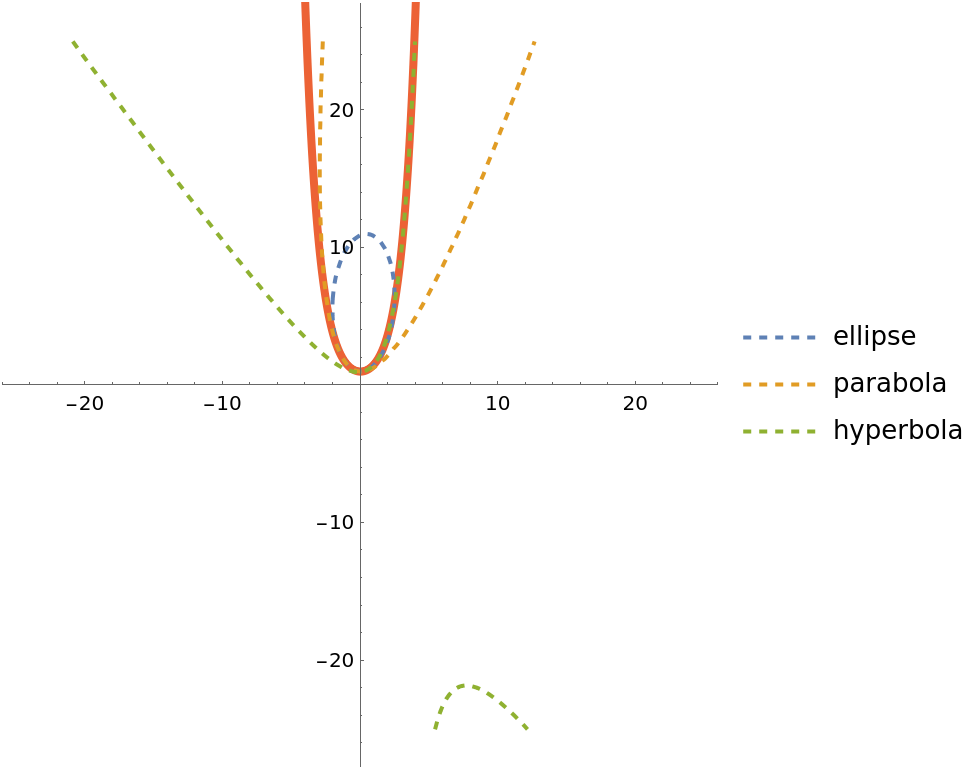### Properties and Relations (1)

Use the resource function ConicProperties to get further information on an osculating conic:

 In:=Out=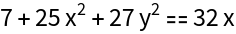In:=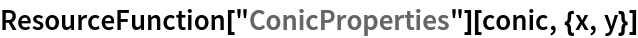Out=## Version History

• 1.0.0 – 08 March 2021